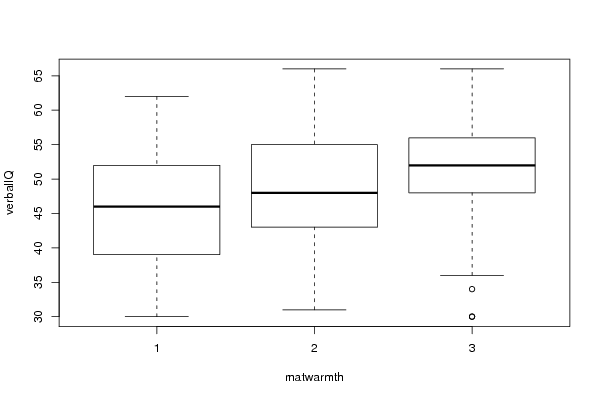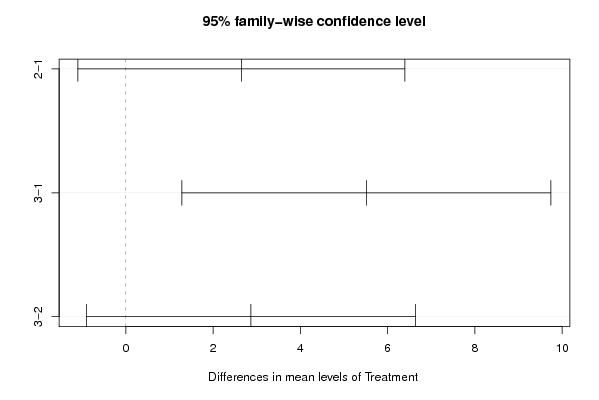## Free Statistics

of Irreproducible Research!

Author's title
Author*The author of this computation has been verified*
R Software ModuleIan.Hollidayrwasp_One Factor ANOVA.wasp
Title produced by softwareChi Square Measure of Association- Free Statistics Software (Calculator)
Date of computationThu, 10 Dec 2009 14:30:01 +0100
Cite this page as followsStatistical Computations at FreeStatistics.org, Office for Research Development and Education, URL https://freestatistics.org/blog/index.php?v=date/2009/Dec/10/t12604518424cd3ujl2adfvlai.htm/, Retrieved Wed, 04 Oct 2023 00:43:21 +0000
Statistical Computations at FreeStatistics.org, Office for Research Development and Education, URL https://freestatistics.org/blog/index.php?pk=65346, Retrieved Wed, 04 Oct 2023 00:43:21 +0000
QR Codes:Original text written by user:
IsPrivate?No (this computation is public)
User-defined keywords
Estimated Impact155
Family? (F = Feedback message, R = changed R code, M = changed R Module, P = changed Parameters, D = changed Data)
-     [Chi Square Measure of Association- Free Statistics Software (Calculator)] [One Way ANOVA wit...] [2009-11-29 13:09:19] [98fd0e87c3eb04e0cc2efde01dbafab6]
-   PD  [Chi Square Measure of Association- Free Statistics Software (Calculator)] [One Way ANOVA for...] [2009-12-01 13:05:10] [3fdd735c61ad38cbc9b3393dc997cdb7]
- R P     [Chi Square Measure of Association- Free Statistics Software (Calculator)] [CARE date with Tu...] [2009-12-01 18:33:48] [98fd0e87c3eb04e0cc2efde01dbafab6]
-   PD      [Chi Square Measure of Association- Free Statistics Software (Calculator)] [comp9, 1] [2009-12-03 13:29:22] [e8bb49267f0b4e611f4778412d0811f2]
-    D        [Chi Square Measure of Association- Free Statistics Software (Calculator)] [comp 9, 2] [2009-12-03 13:38:11] [e8bb49267f0b4e611f4778412d0811f2]
-    D          [Chi Square Measure of Association- Free Statistics Software (Calculator)] [comp 3, 3] [2009-12-03 13:47:54] [e8bb49267f0b4e611f4778412d0811f2]
-    D            [Chi Square Measure of Association- Free Statistics Software (Calculator)] [comp 3, 4] [2009-12-03 13:50:36] [e8bb49267f0b4e611f4778412d0811f2]
-    D                [Chi Square Measure of Association- Free Statistics Software (Calculator)] [comp 9, 5] [2009-12-10 13:30:01] [5b58052098f4b0433c56ae9d9a4453be] [Current]
-    D                  [Chi Square Measure of Association- Free Statistics Software (Calculator)] [comp 9, 6] [2009-12-10 13:34:41] [e8bb49267f0b4e611f4778412d0811f2]
-    D                  [Chi Square Measure of Association- Free Statistics Software (Calculator)] [comp 9, 6] [2009-12-10 13:34:41] [e8bb49267f0b4e611f4778412d0811f2]
-    D                  [Chi Square Measure of Association- Free Statistics Software (Calculator)] [comp 9, 6] [2009-12-10 13:34:41] [e8bb49267f0b4e611f4778412d0811f2]
Feedback Forum

Post a new message
Dataseries X:
36	1
36	1
56	2
48	2
32	2
44	1
39	2
34	2
41	3
50	3
39	1
62	3
52	2
37	3
50	2
41	1
55	2
41	2
56	3
39	2
52	1
46	2
44	2
48	2
41	2
50	3
50	3
44	2
52	1
54	2
44	2
52	3
37	2
52	3
50	3
36	1
50	1
52	3
55	3
31	2
36	1
49	1
42	1
37	2
41	2
30	1
52	1
30	3
41	2
44	1
66	2
48	3
43	2
57	2
46	1
54	3
48	3
48	2
52	1
62	1
58	3
58	2
62	2
48	2
46	2
34	1
66	2
52	3
55	2
55	1
57	3
56	1
55	2
56	3
54	1
55	2
46	2
52	1
32	2
44	1
46	2
59	2
46	3
46	3
54	3
66	3
56	2
59	2
57	2
52	3
48	1
44	1
41	2
50	1
48	3
48	2
59	2
46	2
54	1
55	2
54	3
59	2
44	2
54	3
52	3
66	3
44	2
57	2
39	1
60	3
45	2
41	2
50	2
39	2
43	2
48	1
37	2
58	2
46	1
43	1
44	2
34	3
30	1
50	3
39	1
37	2
55	2
48	3
39	1
36	3
43	2
50	3
55	2
43	2
60	3
48	2
30	3
43	2
39	1
52	2
39	1
39	1
56	1
59	1
46	2
57	2
50	2
54	1
50	3
60	3
59	3
41	2
48	1
59	2
60	3
56	2
56	2
51	1


 Summary of computational transaction Raw Input view raw input (R code) Raw Output view raw output of R engine Computing time 3 seconds R Server 'Gwilym Jenkins' @ 72.249.127.135

\begin{tabular}{lllllllll}
\hline
Summary of computational transaction \tabularnewline
Raw Input & view raw input (R code)  \tabularnewline
Raw Output & view raw output of R engine  \tabularnewline
Computing time & 3 seconds \tabularnewline
R Server & 'Gwilym Jenkins' @ 72.249.127.135 \tabularnewline
\hline
\end{tabular}
%Source: https://freestatistics.org/blog/index.php?pk=65346&T=0

[TABLE]
[ROW][C]Summary of computational transaction[/C][/ROW]
[ROW][C]Raw Input[/C][C]view raw input (R code) [/C][/ROW]
[ROW][C]Raw Output[/C][C]view raw output of R engine [/C][/ROW]
[ROW][C]Computing time[/C][C]3 seconds[/C][/ROW]
[ROW][C]R Server[/C][C]'Gwilym Jenkins' @ 72.249.127.135[/C][/ROW]
[/TABLE]
Source: https://freestatistics.org/blog/index.php?pk=65346&T=0

Globally Unique Identifier (entire table): ba.freestatistics.org/blog/index.php?pk=65346&T=0

As an alternative you can also use a QR Code:

The GUIDs for individual cells are displayed in the table below:

 Summary of computational transaction Raw Input view raw input (R code) Raw Output view raw output of R engine Computing time 3 seconds R Server 'Gwilym Jenkins' @ 72.249.127.135

 ANOVA Model verbalIQ ~ matwarmth means 45.558 2.647 5.513

\begin{tabular}{lllllllll}
\hline
ANOVA Model \tabularnewline
verbalIQ  ~  matwarmth \tabularnewline
means & 45.558 & 2.647 & 5.513 \tabularnewline
\hline
\end{tabular}
%Source: https://freestatistics.org/blog/index.php?pk=65346&T=1

[TABLE]
[ROW][C]ANOVA Model[/C][/ROW]
[ROW][C]verbalIQ  ~  matwarmth[/C][/ROW]
[ROW][C]means[/C][C]45.558[/C][C]2.647[/C][C]5.513[/C][/ROW]
[/TABLE]
Source: https://freestatistics.org/blog/index.php?pk=65346&T=1

Globally Unique Identifier (entire table): ba.freestatistics.org/blog/index.php?pk=65346&T=1

As an alternative you can also use a QR Code:

The GUIDs for individual cells are displayed in the table below:

 ANOVA Model verbalIQ ~ matwarmth means 45.558 2.647 5.513

 ANOVA Statistics Df Sum Sq Mean Sq F value Pr(>F) matwarmth 2 646.065 323.033 4.76 0.01 Residuals 155 10519.308 67.867

\begin{tabular}{lllllllll}
\hline
ANOVA Statistics \tabularnewline
& Df & Sum Sq & Mean Sq & F value & Pr(>F) \tabularnewline
matwarmth & 2 & 646.065 & 323.033 & 4.76 & 0.01 \tabularnewline
Residuals & 155 & 10519.308 & 67.867 &   &   \tabularnewline
\hline
\end{tabular}
%Source: https://freestatistics.org/blog/index.php?pk=65346&T=2

[TABLE]
[ROW][C]ANOVA Statistics[/C][/ROW]
[ROW][C] [/C][C]Df[/C][C]Sum Sq[/C][C]Mean Sq[/C][C]F value[/C][C]Pr(>F)[/C][/ROW]
[ROW][C]matwarmth[/C][C]2[/C][C]646.065[/C][C]323.033[/C][C]4.76[/C][C]0.01[/C][/ROW]
[ROW][C]Residuals[/C][C]155[/C][C]10519.308[/C][C]67.867[/C][C] [/C][C] [/C][/ROW]
[/TABLE]
Source: https://freestatistics.org/blog/index.php?pk=65346&T=2

Globally Unique Identifier (entire table): ba.freestatistics.org/blog/index.php?pk=65346&T=2

As an alternative you can also use a QR Code:

The GUIDs for individual cells are displayed in the table below:

 ANOVA Statistics Df Sum Sq Mean Sq F value Pr(>F) matwarmth 2 646.065 323.033 4.76 0.01 Residuals 155 10519.308 67.867

 Tukey Honest Significant Difference Comparisons diff lwr upr p adj 2-1 2.647 -1.1 6.395 0.219 3-1 5.513 1.284 9.743 0.007 3-2 2.866 -0.91 6.642 0.174

\begin{tabular}{lllllllll}
\hline
Tukey Honest Significant Difference Comparisons \tabularnewline
& diff & lwr & upr & p adj \tabularnewline
2-1 & 2.647 & -1.1 & 6.395 & 0.219 \tabularnewline
3-1 & 5.513 & 1.284 & 9.743 & 0.007 \tabularnewline
3-2 & 2.866 & -0.91 & 6.642 & 0.174 \tabularnewline
\hline
\end{tabular}
%Source: https://freestatistics.org/blog/index.php?pk=65346&T=3

[TABLE]
[ROW][C]Tukey Honest Significant Difference Comparisons[/C][/ROW]
[ROW][C]2-1[/C][C]2.647[/C][C]-1.1[/C][C]6.395[/C][C]0.219[/C][/ROW]
[ROW][C]3-1[/C][C]5.513[/C][C]1.284[/C][C]9.743[/C][C]0.007[/C][/ROW]
[ROW][C]3-2[/C][C]2.866[/C][C]-0.91[/C][C]6.642[/C][C]0.174[/C][/ROW]
[/TABLE]
Source: https://freestatistics.org/blog/index.php?pk=65346&T=3

Globally Unique Identifier (entire table): ba.freestatistics.org/blog/index.php?pk=65346&T=3

As an alternative you can also use a QR Code:

The GUIDs for individual cells are displayed in the table below:

 Tukey Honest Significant Difference Comparisons diff lwr upr p adj 2-1 2.647 -1.1 6.395 0.219 3-1 5.513 1.284 9.743 0.007 3-2 2.866 -0.91 6.642 0.174

 Levenes Test for Homogeneity of Variance Df F value Pr(>F) Group 2 0.521 0.595 155

\begin{tabular}{lllllllll}
\hline
Levenes Test for Homogeneity of Variance \tabularnewline
& Df & F value & Pr(>F) \tabularnewline
Group & 2 & 0.521 & 0.595 \tabularnewline
& 155 &   &   \tabularnewline
\hline
\end{tabular}
%Source: https://freestatistics.org/blog/index.php?pk=65346&T=4

[TABLE]
[ROW][C]Levenes Test for Homogeneity of Variance[/C][/ROW]
[ROW][C] [/C][C]Df[/C][C]F value[/C][C]Pr(>F)[/C][/ROW]
[ROW][C]Group[/C][C]2[/C][C]0.521[/C][C]0.595[/C][/ROW]
[ROW][C] [/C][C]155[/C][C] [/C][C] [/C][/ROW]
[/TABLE]
Source: https://freestatistics.org/blog/index.php?pk=65346&T=4

Globally Unique Identifier (entire table): ba.freestatistics.org/blog/index.php?pk=65346&T=4

As an alternative you can also use a QR Code:

The GUIDs for individual cells are displayed in the table below:

 Levenes Test for Homogeneity of Variance Df F value Pr(>F) Group 2 0.521 0.595 155PNG link Postscript link PDF linkPNG link Postscript link PDF link

Parameters (Session):
par1 = 1 ; par2 = 2 ; par3 = TRUE ;
Parameters (R input):
par1 = 1 ; par2 = 2 ; par3 = TRUE ;
R code (references can be found in the software module):
cat1 <- as.numeric(par1) #cat2<- as.numeric(par2) #intercept<-as.logical(par3)x <- t(x)x1<-as.numeric(x[,cat1])f1<-as.character(x[,cat2])xdf<-data.frame(x1,f1)(V1<-dimnames(y)[][cat1])(V2<-dimnames(y)[][cat2])names(xdf)<-c('Response', 'Treatment')if(intercept == FALSE) (lmxdf<-lm(Response ~ Treatment - 1, data = xdf) ) else (lmxdf<-lm(Response ~ Treatment, data = xdf) )(aov.xdf<-aov(lmxdf) )(anova.xdf<-anova(lmxdf) )load(file='createtable')a<-table.start()a<-table.row.start(a)a<-table.element(a,'ANOVA Model', length(lmxdf$coefficients)+1,TRUE)a<-table.row.end(a)a<-table.row.start(a)a<-table.element(a, paste(V1, ' ~ ', V2), length(lmxdf$coefficients)+1,TRUE)a<-table.row.end(a)a<-table.row.start(a)a<-table.element(a, 'means',,TRUE)for(i in 1:length(lmxdf$coefficients)){a<-table.element(a, round(lmxdf$coefficients[i], digits=3),,FALSE)}a<-table.row.end(a)a<-table.end(a)table.save(a,file='mytable.tab')a<-table.start()a<-table.row.start(a)a<-table.element(a,'ANOVA Statistics', 5+1,TRUE)a<-table.row.end(a)a<-table.row.start(a)a<-table.element(a, ' ',,TRUE)a<-table.element(a, 'Df',,FALSE)a<-table.element(a, 'Sum Sq',,FALSE)a<-table.element(a, 'Mean Sq',,FALSE)a<-table.element(a, 'F value',,FALSE)a<-table.element(a, 'Pr(>F)',,FALSE)a<-table.row.end(a)a<-table.row.start(a)a<-table.element(a, V2,,TRUE)a<-table.element(a, anova.xdf$Df,,FALSE)a<-table.element(a, round(anova.xdf$'Sum Sq', digits=3),,FALSE)a<-table.element(a, round(anova.xdf$'Mean Sq', digits=3),,FALSE)a<-table.element(a, round(anova.xdf$'F value', digits=3),,FALSE)a<-table.element(a, round(anova.xdf$'Pr(>F)', digits=3),,FALSE)a<-table.row.end(a)a<-table.row.start(a)a<-table.element(a, 'Residuals',,TRUE)a<-table.element(a, anova.xdf$Df,,FALSE)a<-table.element(a, round(anova.xdf$'Sum Sq', digits=3),,FALSE)a<-table.element(a, round(anova.xdf$'Mean Sq', digits=3),,FALSE)a<-table.element(a, ' ',,FALSE)a<-table.element(a, ' ',,FALSE)a<-table.row.end(a)a<-table.end(a)table.save(a,file='mytable1.tab')bitmap(file='anovaplot.png')boxplot(Response ~ Treatment, data=xdf, xlab=V2, ylab=V1)dev.off()if(intercept==TRUE){thsd<-TukeyHSD(aov.xdf)bitmap(file='TukeyHSDPlot.png')plot(thsd)dev.off()a<-table.start()a<-table.row.start(a)a<-table.element(a,'Tukey Honest Significant Difference Comparisons', 5,TRUE)a<-table.row.end(a)a<-table.row.start(a)a<-table.element(a, ' ', 1, TRUE)for(i in 1:4){a<-table.element(a,colnames(thsd[])[i], 1, TRUE)}a<-table.row.end(a)for(i in 1:length(rownames(thsd[]))){a<-table.row.start(a)a<-table.element(a,rownames(thsd[])[i], 1, TRUE)for(j in 1:4){a<-table.element(a,round(thsd[][i,j], digits=3), 1, FALSE)}a<-table.row.end(a)}a<-table.end(a)table.save(a,file='mytable2.tab')}if(intercept==FALSE){a<-table.start()a<-table.row.start(a)a<-table.element(a,'TukeyHSD Message', 1,TRUE)a<-table.row.end(a)a<-table.start()a<-table.row.start(a)a<-table.element(a,'Must Include Intercept to use Tukey Test ', 1, FALSE)a<-table.row.end(a)a<-table.end(a)table.save(a,file='mytable2.tab')}library(car)lt.lmxdf<-levene.test(lmxdf)a<-table.start()a<-table.row.start(a)a<-table.element(a,'Levenes Test for Homogeneity of Variance', 4,TRUE)a<-table.row.end(a)a<-table.row.start(a)a<-table.element(a,' ', 1, TRUE)for (i in 1:3){a<-table.element(a,names(lt.lmxdf)[i], 1, FALSE)}a<-table.row.end(a)a<-table.row.start(a)a<-table.element(a,'Group', 1, TRUE)for (i in 1:3){a<-table.element(a,round(lt.lmxdf[[i]], digits=3), 1, FALSE)}a<-table.row.end(a)a<-table.row.start(a)a<-table.element(a,' ', 1, TRUE)a<-table.element(a,lt.lmxdf[], 1, FALSE)a<-table.element(a,' ', 1, FALSE)a<-table.element(a,' ', 1, FALSE)a<-table.row.end(a)a<-table.end(a)table.save(a,file='mytable3.tab')